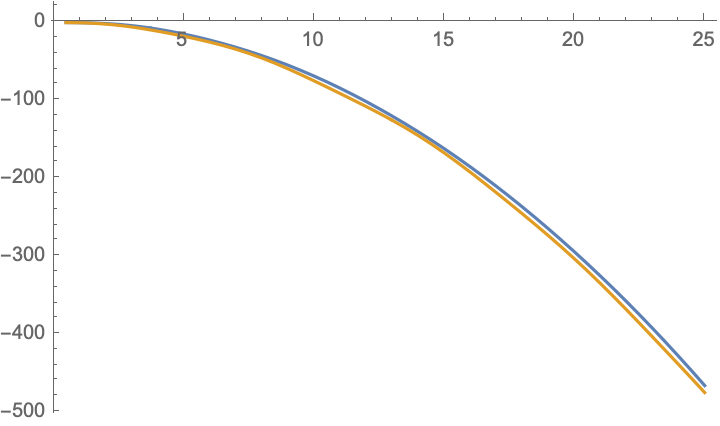# Sum of $\sin$ when angles shrink by $1/n$

There are many identities known like

$$\sum_{k=0}^{n-1} \sin (k \cdot \theta + \varphi) = \frac{\sin\left(n \cdot \frac{\theta}{2}\right)}{\sin\left(\frac{\theta}{2}\right)} \cdot \sin \left(\frac{2 \varphi + (n-1)\cdot \theta}{2} \right)$$

However, in such situations the angles add up and are equidistant. Instead, I pose myself the question whether there is a simplifying formula for $$\sum_{k=1}^{n} k \cdot \sin \left(\frac{x}{k} \right),$$ particularly, if $$x$$ is much larger than $$n$$.

• The original version is basically asking for $\sum_{k=1}^n \sin\frac xk$ where $k=n\theta+\varphi$. I just wanted to make sure whether you meant $\sin \left(\frac{n \cdot \theta + \varphi}{k} \right)$ or $\sin \left(\frac{k \cdot \theta + \varphi}{k} \right)$. Jan 17, 2021 at 10:15
• After trying SearchOnMath and ApproachZero, I found this question: Estimating $\sum\limits _{n=1}^k \sin \frac x n$ in the form $f(k,x) \sin(g(k,x))$. Jan 17, 2021 at 10:22
• @Martin There was indeed an error in the old formula, but I had to add a factor of k to it Jan 17, 2021 at 10:47
• So, the title, which says "angles shrink by $1/n$"? Jan 17, 2021 at 11:23

For large $$n$$ you may approximate the sum by an integral, which gives $$\sum_{k=1}^{n} k \sin (x/k)-nx\simeq \int_0^\infty\bigl(k\sin(x/k)-k\bigr)\,dk=-\tfrac{1}{4}\pi x^2.$$ The plot compares the left-hand-side of this equation for $$n=1000$$ (blue curve) with the right-hand-side (gold) as a function of $$x$$.• Many thanks for your input. I like your formula a lot, however, I am interested in situations where $x$ is much larger than $n$ and I am afraid that this is not going to hold anymore then. Or is there a way to improve that formula? Jan 17, 2021 at 22:13
If you rewrite everything using complex exponentials, then it's clear why a sum such as $$\sum_{k=0}^{n-1}\sin(k\theta+\phi)$$ has a nice closed formula, since it's just two geometric sums $$e^{i\phi}\sum_{k=1}^{n-1} e^{\pm i\theta k}.$$ And if you want $$\sum_{k=0}^{n-1}k\sin(k\theta+\phi)$$, it's similar, there are nice formulas for $$\sum_{k=1}^{n-1}kT^k$$. On the other hand, the sum you're asking about looks like $$\sum_{k=1}^n k\sin\left(\frac{x}{k}\right) = \frac12\sum_{k=1}^n \Bigl(ke^{\pi i x/k} + ke^{-\pi i x/k}\Bigr).$$ I don't think that there's a nice closed formula in general for $$\sum_{k=1}^n kT^{1/k},\quad\text{nor for}\quad \sum_{k=1}^n T^{1/k}.$$ However, as Carlo indicated, one can often get a good approximation using an integral.